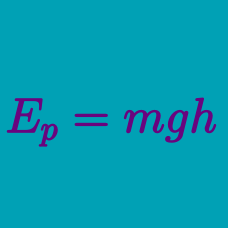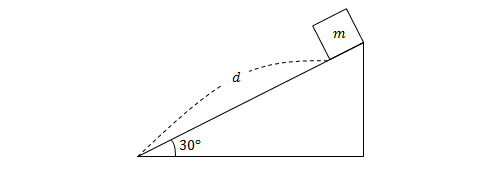Classical Mechanics

Potential energy due to gravityA crate of mass $m=4\text{ kg}$ slides down a frictionless inclined plane of length $d=8\text{ m}$ that makes a $30^\circ$ angle with the horizontal. What is the crate's change in gravitational potential energy when it reaches the bottom of the inclined plane?

The gravitational acceleration is $g=10\text{ m/s}^2.$

A $6\text{ kg}$ object is dropped from the edge of a $150\text{ m}$ high cliff. What is its gravitational potential energy (relative to the ground) after $3$ seconds?

Ignore any air resistance and assume that gravitational acceleration is $g=10\text{ m/s}^2.$

A $80\text{ kg}$ man stands on the edge of a $50$-meter-high cliff. What is his gravitational potential energy relative to the ground?

The gravitational acceleration is $g=10\text{ m/s}^2.$

An old English nursery rhyme starts off "Jack and Jill went up the hill to fetch a pail of water". If the hill is 10 m high and Jack weighs 700 N, how much work in Joules did Jack need to do to get to the top of the hill?

An object of mass $3\text{ kg}$ is dropped from a height of $102\text{ m}.$ After $2$ seconds, the object's gravitational potential energy is $E_1,$ and $4$ seconds after the drop it is $E_2.$ Find the value of $E_1+E_2.$

The air resistance is negligible and the gravitational acceleration is $g=10\text{ m/s}^2.$

×